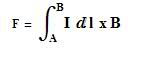# Current in a Wire.

wakejosh

## Homework Statement

The force exerted on a current carrying wire located in an external magnetic field is directly proportional to which of the following?
a. current in the wire
b. magnetic field strength
c. length of the wire
d. answers a, b and c are all correct
e. none of the above are correct

## The Attempt at a Solution

pretty sure its D. just wanted to get a second opinion. thanks.

## Answers and Replies

Galileo_Galilei
Yo man, that sounds like an IB paper 1 question.

You doing IB Physics too?

Metaleer
wakejosh, the force exerted on a current carrying wire located in an external magnetic field is given by the following expression:What does that tell you? :)

wakejosh
well, it tells me that A and B are correct, but i'm just not totally sure if C is correct, but since I dont have any options to say that A and B are correct, then I am thinking D has to be the correct answer, and that wire length is also important.

mbrmbrg
Good use of logic skills there!

But the integral of dl is length... so the equation does tell you about length.

Metaleer
wakejosh, the thing is when you integrate between "A" and "B", what you get is the length (of course, if both the current intensity and magnetic field don't depend on the length, you can simply extract them from the antiderivative and when you integrate, you simply get the length).